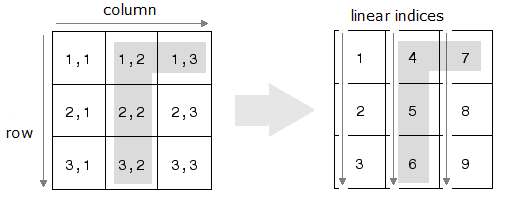# sub2ind

Convert subscripts to linear indices

## Syntax

``ind = sub2ind(sz,row,col)``
``ind = sub2ind(sz,I1,I2,...,In)``

## Description

example

````ind = sub2ind(sz,row,col)` returns the linear indices `ind` corresponding to the row and column subscripts in `row` and `col` for a matrix of size `sz`. Here `sz` is a vector with two elements, where `sz(1)` specifies the number of rows and `sz(2)` specifies the number of columns. ```

example

````ind = sub2ind(sz,I1,I2,...,In)` returns the linear indices `ind` corresponding to multidimensional subscripts in `n` arrays `I1,I2,...,In` for a multidimensional array of size `sz`. Here `sz` is a vector with `n` elements that specifies the size of each array dimension.```

## Examples

collapse all

The mapping from subscripts (indexing by position) to linear indices for a 3-by-3 matrix can be illustrated as in the following.Specify the row and column subscripts in a 3-by-3 matrix. Convert the subscripts to linear indices.

```row = [1 2 3 1]; col = [2 2 2 3]; sz = [3 3]; ind = sub2ind(sz,row,col)```
```ind = 1×4 4 5 6 7 ```

The mapping from subscripts to linear indices for a 2-by-2-by-2 array can be illustrated as in the following.Specify the row, column, and page subscripts in a 2-by-2-by-2 array. Convert the subscripts to linear indices.

```I1 = [1 2 1 2]; I2 = [2 2 1 1]; I3 = [1 1 2 2]; sz = [2 2 2]; ind = sub2ind(sz,I1,I2,I3)```
```ind = 1×4 3 4 5 6 ```

Convert a subscript index of a 3-D array to a single linear index.

Create an array, and find the linear index corresponding to the element in the (2,1,2) position.

```A = rand(3,4,2); linearInd = sub2ind(size(A),2,1,2)```
```linearInd = 14 ```

Check that both index versions refer to the same element.

`A(2,1,2)`
```ans = 0.4854 ```
`A(14)`
```ans = 0.4854 ```

## Input Arguments

collapse all

Size of array, specified as a vector of positive integers. Each element of this vector indicates the size of the corresponding dimension. For example, ```[2 3 4]``` defines a 2-by-3-by-4 array.

Data Types: `single` | `double` | `int8` | `int16` | `int32` | `int64` | `uint8` | `uint16` | `uint32` | `uint64`

Row subscripts, specified as a scalar, vector, matrix, or multidimensional array. The size of `row` must be the same as the size of `col`.

Data Types: `single` | `double` | `int8` | `int16` | `int32` | `int64` | `uint8` | `uint16` | `uint32` | `uint64`

Column subscripts, specified as a scalar, vector, matrix, or multidimensional array. The size of `col` must be the same as the size of `row`.

Data Types: `single` | `double` | `int8` | `int16` | `int32` | `int64` | `uint8` | `uint16` | `uint32` | `uint64`

Multidimensional subscripts, specified as a scalar, vector, matrix, or multidimensional array. Each array `I1,I2,…,In` must have the same size.

Data Types: `single` | `double` | `int8` | `int16` | `int32` | `int64` | `uint8` | `uint16` | `uint32` | `uint64`

## Output Arguments

collapse all

Linear indices, specified as a scalar, vector, matrix, or multidimensional array. The size of `ind` is the same as the size of the input `row`, `col`, or `I1,I2,…,In`.

Data Types: `double`

## Algorithms

For an array `A`, if `ind = sub2ind(size(A),I1,…,In)`, then `A(ind(k)) = A(I1(k),…,In(k))` for all `k`.

## Version History

Introduced before R2006a